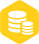# 八步设计反激式开关电源变压器

### 描述I升=VS*Ton/L

Ｉ降=VOR*TOFF/L

VS*TON/L=VOR*TOFF/L

D= VOR/（VOR +VS）

Iav=Po/（η*VS）S=IM*KRP*D/2+IM*（1-KRP）*D

IM=Iav/[(1-0.5 KRP）*D]。

Iav=10/0.8*90=0.138A,

IM=0.138/(1-0.5KRP).D=0.138/(1-0.5*0.6)*0.47=0.419A.NP=VS*TON/SJ*B

NS=NP*(UO+UF)/VOR,

L=VS.TON/(IM*KRP)×
20